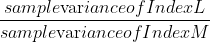Scan QR code or get instant email to install app

Question:

# An analyst is testing the hypothesis that the variance of monthly returns for Index L equals the variance of monthly returns for Index M based on samples of 50 monthly observations. The sample variance of Index L returns is 0.085, whereas the sample variance of Index M returns is 0.084. Assuming the samples are independent and the returns are normally distributed, which of the following represents the most appropriate test statistic?

A 1212explanation

The appropriate test is an .F-test, where the larger sample variance (Index L) is placed in the numerator.